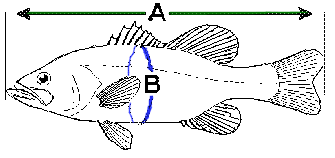# Estimating fish weight

## Fishing Wisconsin

Fish are sometimes damaged when weighed. Using the appropriate formula for your species below, you can quickly calculate the "approximate" weight of your fish by using a ruler. You may also wish to download our trophy converter [PDF] in which we've done the math for you.

Please note when using the formulas:

• length and girth are measured in inches,
• girth is the distance around the body of the fish at its largest point, and
• all answers are in poundsA = length and B = girth

## Formulas

bass weight = (length x length x girth) / 1,200

pike weight = (length x length x length) / 3,500

sunfish weight = (length x length x length) / 1,200

trout weight = (length x girth x girth) / 800

walleye weight = (length x length x length) / 2,700

For example, you catch a 16 inch walleye. Using the formula for walleye above: (16 x 16 x 16) / 2,700 = 1.5 pounds. Your walleye would weigh approximately 1.5 pounds.